# SUBTOTAL function: Calculate the data with the hidden value### Graphic skills

Take this table as an example. When using the SUM function to do summations, we may find two rows hidden in the table, but the result of the summation still includes the hidden value.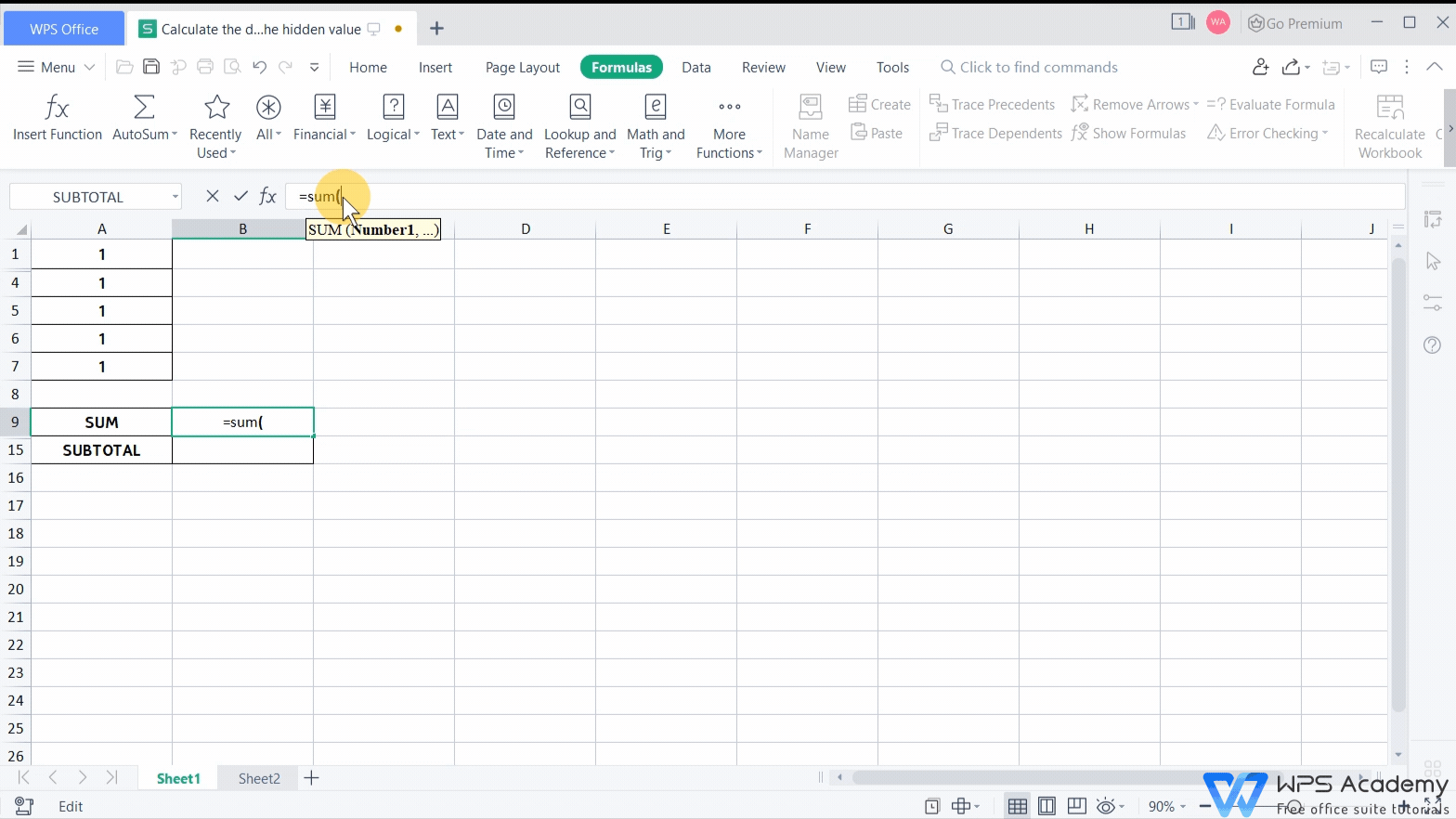So how can we calculate the data containing hidden values and get a result that does not include the hidden values? This video can help us and it can be also used in Microsoft Office Excel and Openoffice.

In this case, we can use the SUBTOTAL function. Next, we are going to show you the usage of the SUBTOTAL function. Its corresponding function code is also shown in the table in this video.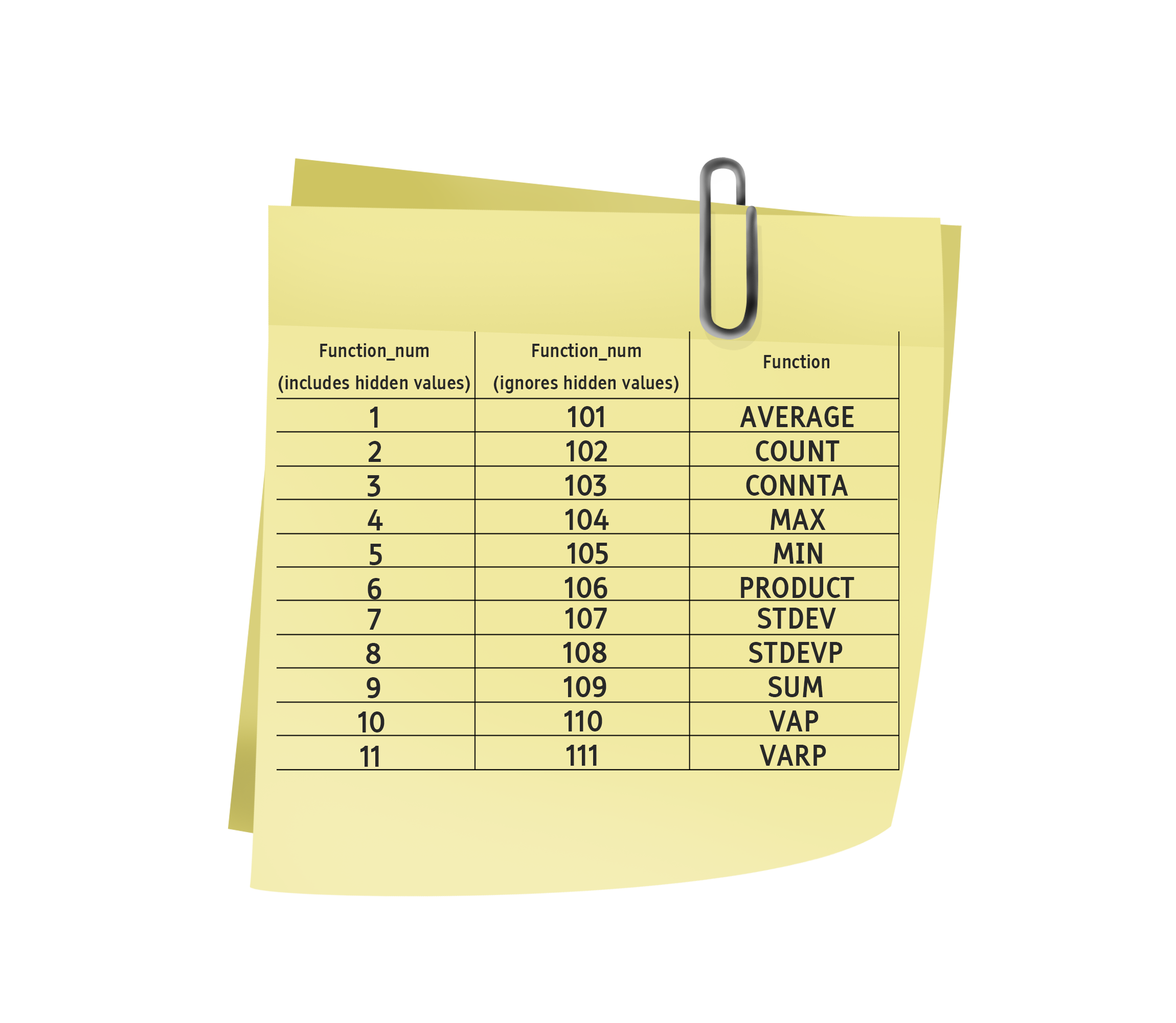1. Click to insert the SUBTOTAL function.

2. Enter the function code 109 of ignores hidden values of SUM function in the function number.

3. Select the cell range A1: A7 in the reference, and click OK to get the result.

At this time, the calculation result will not include the hidden cell data. When we hide other data, the calculation result will change accordingly. That is to say, only the data of the display unit will be calculated.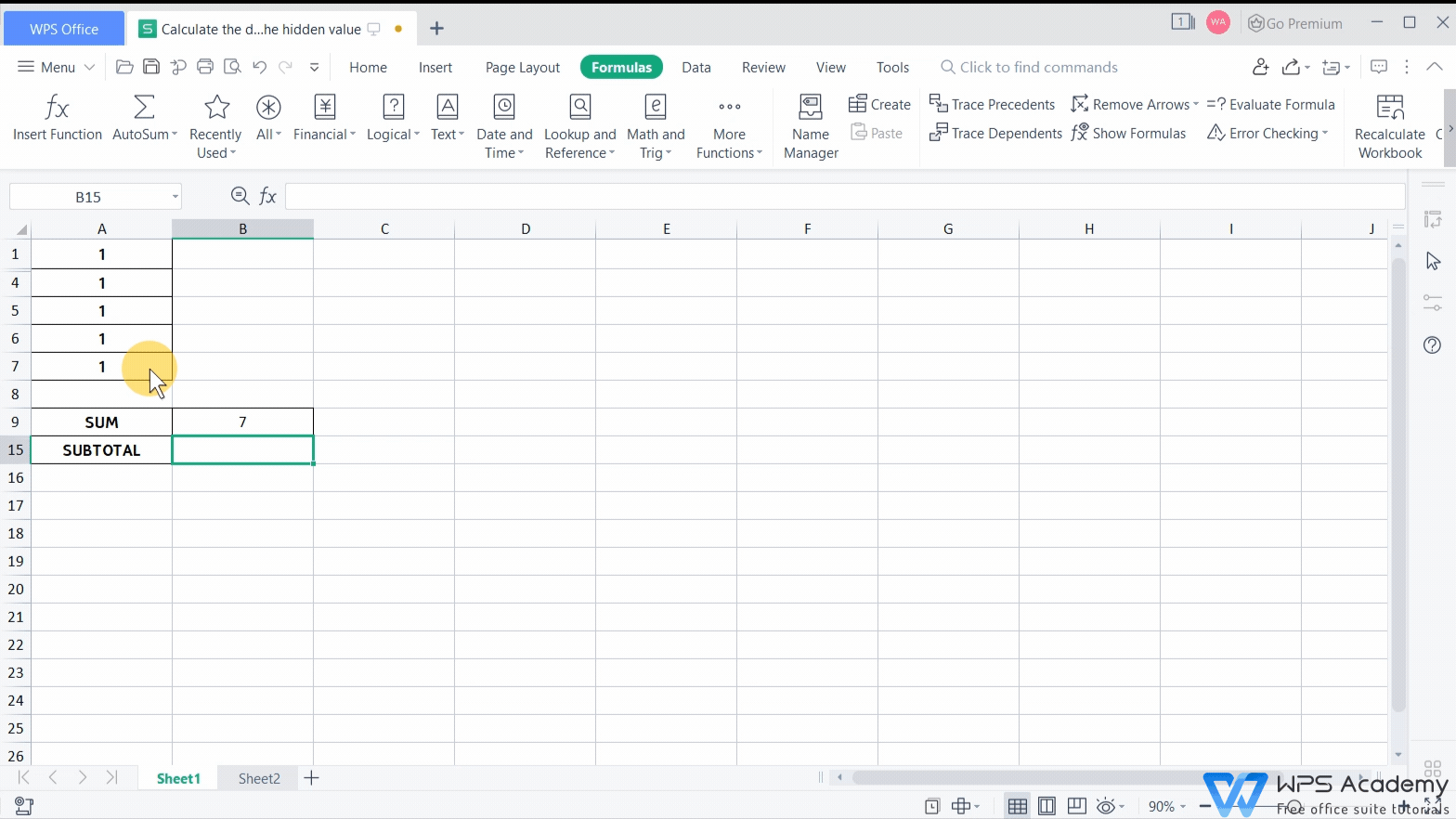In addition, we can also use the SUBTOTAL function in many scenarios.

Take sheet 2 as an example. These are the grades of some students in a study group. Now we need to calculate the average grade of this study group. To make the result closer to the actual situation, we usually choose to ignore the highest and lowest data.

1. Now, we only need to click to insert the SUBTOTAL function.

2. Enter the corresponding AVERAGE function code 101 and select the reference cell range C3: C9.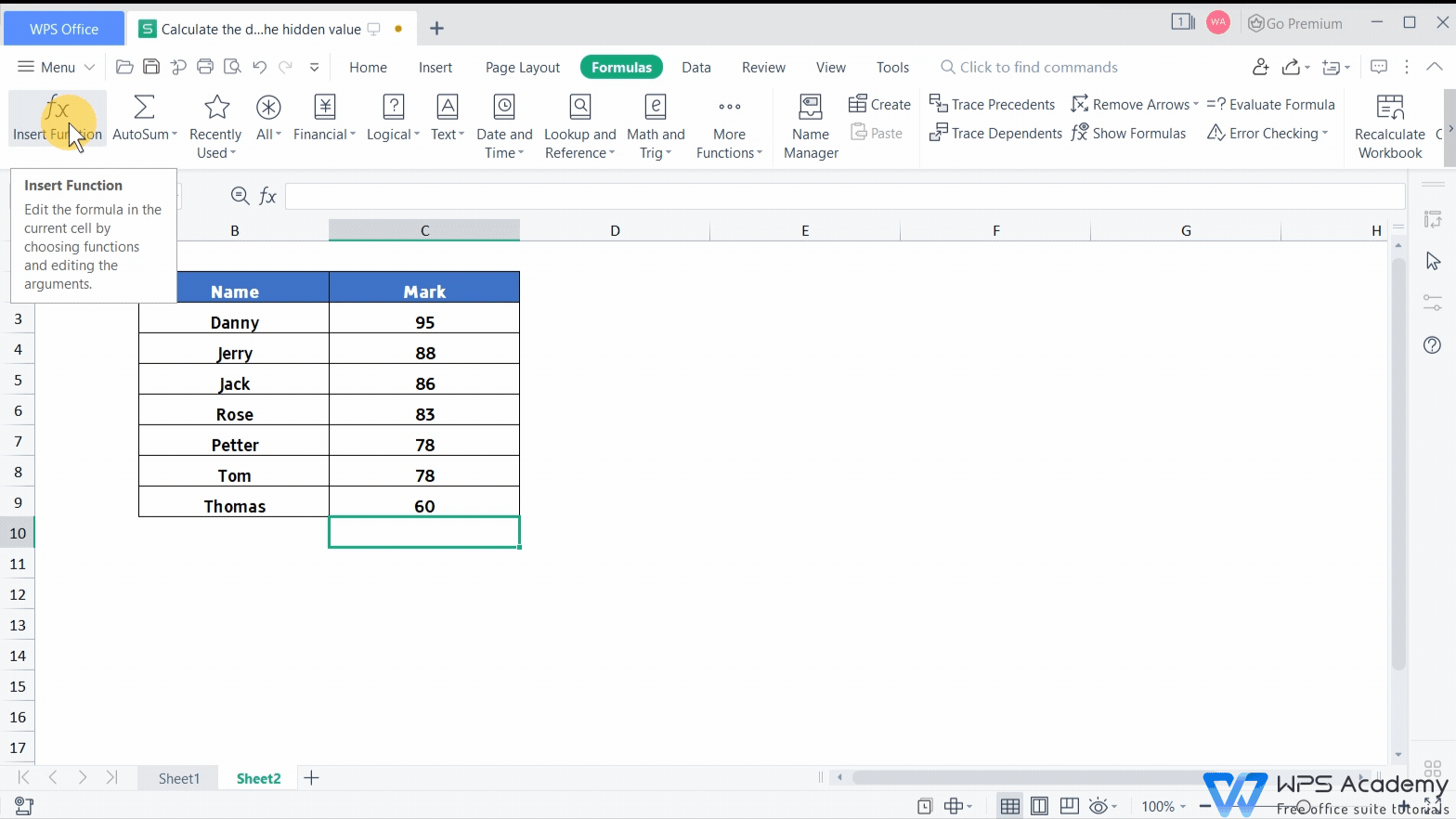3. Finally, click OK. After we get the result, hide the highest and lowest scores.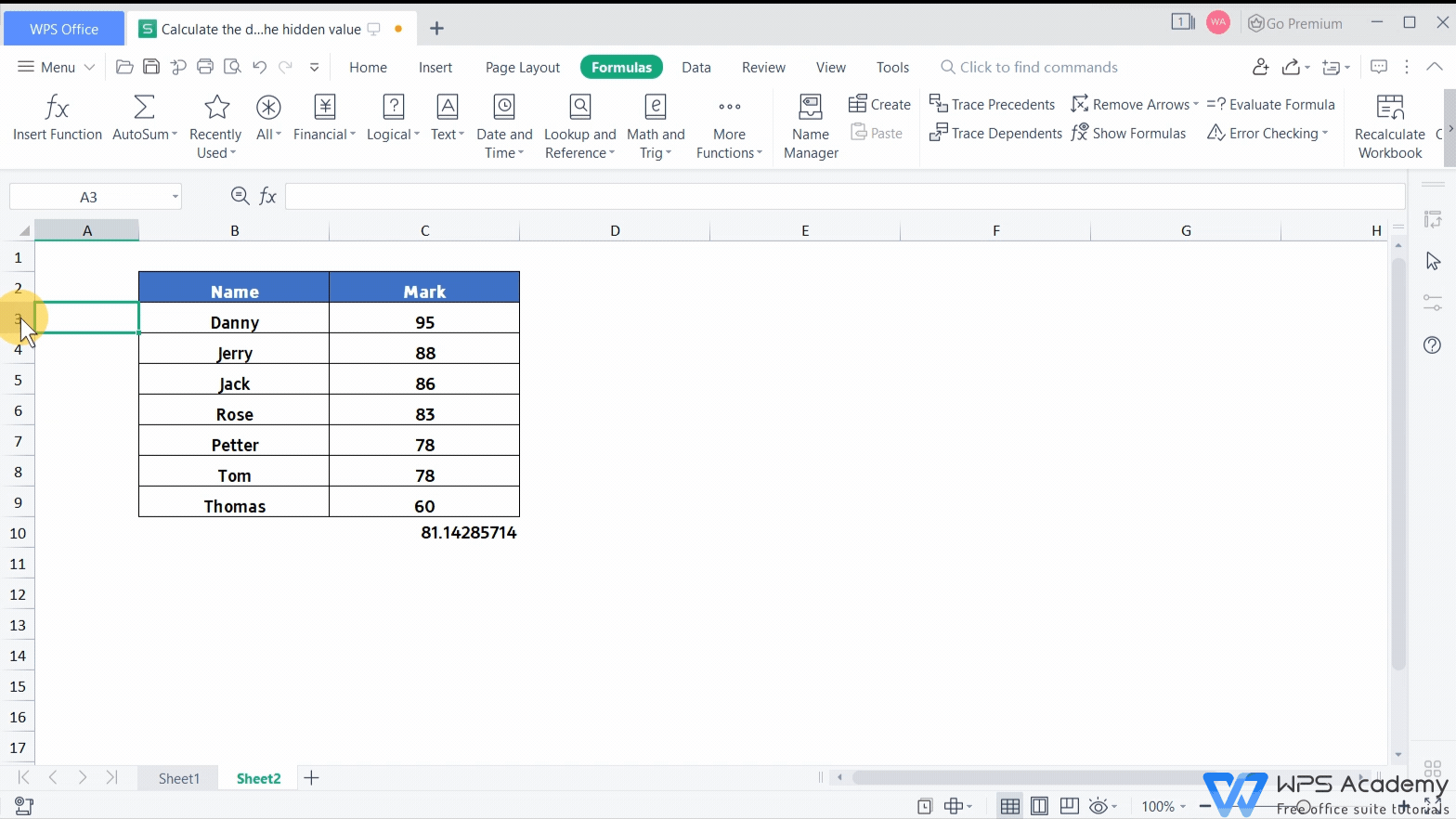SUBTOTAL is often used to calculate the hidden value in the table. When we need to ignore the hidden value of the table for calculation, use the function code in the ignores hidden values column. When we need to include hidden values for calculation, choose the corresponding function code in the includes hidden values column.

This is the introduction on SUBTOTAL function. Did you get it? You are also welcome to join us in our WPS Academy to watch more related videos.